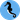# Hippocampus's Garden

Under the sea, in the hippocampus's garden...

# Custom Objective for LightGBM

November 22, 2020  |  7 min read  |  154 views

Gradient boosting decision trees (GBDTs) like XGBoost, LightGBM, and CatBoost are the most popular models in tabular data competitions. These packages come with many built-in objective functions for a variety of use cases. However, sometimes you might want to use a custom objective function that you define yourself. This was somewhat tricky to me when I tried, so I share how to do it in this post.

Specifically, taking the L2 loss and the binary cross-entropy loss for examples, I discuss how to re-implement those loss functions and compare the results from the built-in loss and custom loss. Although I use LightGBM’s Python distribution in this post, essentially the same argument should hold for other packages as well.

The script used in the experiment section is available here. In the following part, I assume you have imported these packages:

import numpy as np
import pandas as pd
import lightgbm as lgb
from sklearn.model_selection import train_test_split
from sklearn.metrics import mean_squared_error, accuracy_score
import seaborn as sns

## Regression (L2 Loss)

Let’s start with the simpler problem: regression. The entire process is three-fold:

1. Calculate the first- and second-order derivatives of the objective function
2. Implement two functions; One returns the derivatives and the other returns the loss itself
3. Specify the defined functions in lgb.train()

### Calculating Derivatives

Like Newton’s method, modern GBDT algorithms utilize the 2nd-order derivative as well as the gradient to create new branches. So, we need to calculate the first- and second-order derivatives. Let $t$ denote the ground truth and $y$ denote the model’s prediction, and we get:

$\mathcal{L}(y,t) = \frac{1}{2}(y-t)^2$ $\frac{\partial \mathcal{L}(y,t)}{\partial y} = y-t$ $\frac{\partial^2 \mathcal{L}(y,t)}{\partial y^2} = 1$

### Implementation

We need to define two functions. One returns the first- and second-order derivatives and is used to train the model. The other is a metric function, which returns the name of the loss function, the loss value, and whether a higher value is desirable or not. This is used for early stopping, learning curve plot, and so on.

def l2_loss(y, data):
t = data.get_label()
hess = np.ones_like(y)

def l2_eval(y, data):
t = data.get_label()
loss = (y - t) ** 2
return 'l2', loss.mean(), False

Note: The second-order derivative that we consider here is a vector of the same shape as the gradient and not a matrix, but it is conventionally called Hessian.

### Comparison to Built-in Objective

Next, I checked if the custom function is valid or not by comparing it to the result from the built-in loss function. Here I used the “tips” dataset as an example.

df = sns.load_dataset('tips')
X_train, X_test, y_train, y_test = train_test_split(df.drop(['total_bill'], axis=1), df['total_bill'], random_state=0)
lgb_train = lgb.Dataset(X_train, y_train)

# Using built-in objective
lgbm_params = {
'objective': 'regression',
'random_seed': 0
}
model = lgb.train(lgbm_params,
lgb_train)
y_pred = model.predict(X_test)
print(mean_squared_error(y_test, y_pred))
# >> 31.94812324773213

# Using custom objective
lgbm_params = {
'random_seed': 0
}

model = lgb.train(lgbm_params,
lgb_train,
fobj=l2_loss,
feval=l2_eval)
y_pred = model.predict(X_test)
print(mean_squared_error(y_test, y_pred))
# >> 31.947398098316526

I obtained the fairly close results, so the functions implemented above should be correct.

### Weighting

You can give different weights to samples by lgb.Dataset(data, label, weight) or something like that. If you want to use them in the custom objective, call data.get_weight() like:

def l2_loss(y, data):
t = data.get_label()
w = data.get_weight()
grad = w * (y - t)
hess = w

def l2_eval(y, data):
t = data.get_label()
w = data.get_weight()
loss = np.sum(w * (y - t)**2) / np.sum(w)
return 'weighted_l2', loss, False

## Binary Classification (Cross-Entropy Loss)

Binary classification is more difficult than regression. First, you should be noted that the model outputs the logit $z$ rather than the probability $y=\mathrm{sigmoid}(z) = 1/(1+e^{-z})$. Second, numerical stability matters when exponential and logarithmic functions are involved.

### Calculating Derivatives

This time, we do the derivative for $z$ instead of $y=\mathrm{sigmoid}(z)$.

\begin{aligned} \mathcal{L}(z,t) &= -t\ln{y}-(1-t)\ln{(1-y)}\\ &= -t\ln{\frac{1}{1+e^{-z}}}-(1-t)\ln{\frac{e^{-z}}{1+e^{-z}}}\\ &=t\ln(1+e^{-z})+(1-t) \ln(1+e^{z}) \\ &= t~\mathrm{softplus}(-z) + (1-t)~\mathrm{softplus}(z) \end{aligned} \begin{aligned} \frac{\partial \mathcal{L}(z,t)}{\partial z} &= -t~\frac{e^{-z}}{1+e^{-z}} + (1-t)~\frac{e^{z}}{1+e^{z}}\\ &= -t~\frac{e^{-z}}{1+e^{-z}} + (1-t)~\frac{1}{1+e^{-z}}\\ &= \frac{1}{1+e^{-z}} - t\\ &= y-t \end{aligned} \begin{aligned} \frac{\partial^2 \mathcal{L}(z,t)}{\partial z^2} &= \frac{\partial}{\partial z}\frac{1}{1+e^{-z}}\\ &= \frac{e^{-z}}{(1+e^{-z})^2}\\ &= y(1-y) \end{aligned}

### Implementation

In reference to this post, I implemented a numerically stable loss function.

def _positive_sigmoid(x):
return 1 / (1 + np.exp(-x))

def _negative_sigmoid(x):
exp = np.exp(x)
return exp / (exp + 1)

def sigmoid(x):
positive = x >= 0
negative = ~positive
result = np.empty_like(x)
result[positive] = _positive_sigmoid(x[positive])
result[negative] = _negative_sigmoid(x[negative])
return result

def _positive_softplus(x):
return x + np.log1p(np.exp(-x))

def _negative_softplus(x):
return np.log1p(np.exp(x))

def softplus(x):
positive = x >= 0
negative = ~positive
result = np.empty_like(x)
result[positive] = _positive_softplus(x[positive])
result[negative] = _negative_softplus(x[negative])
return result

def bce_loss(z, data):
t = data.get_label()
y = sigmoid(z)
hess = y * (1 - y)

def bce_eval(z, data):
t = data.get_label()
loss = t * softplus(-z) + (1 - t) * softplus(z)
return 'bce', loss.mean(), False

### Comparison to Built-in Objective

Again, I checked if the cutom function is valid or not by a simple experiment.

data = load_breast_cancer()
df = pd.DataFrame(data.data, columns=data.feature_names)
X_train, X_test, y_train, y_test = train_test_split(df, data.target, random_state=0)
lgb_train = lgb.Dataset(X_train, y_train)

lgbm_params = {
'objective': 'binary',
'random_seed': 0
}
model = lgb.train(lgbm_params,
lgb_train)
y_pred = model.predict(X_test)
print(accuracy_score(y_test, y_pred>0.5))
# >> 0.972027972027972

lgbm_params = {
'random_seed': 0
}
model = lgb.train(lgbm_params,
lgb_train,
fobj=bce_loss,
feval=bce_eval)
# Note: When using custom objective, the model outputs logits
y_pred = sigmoid(model.predict(X_test))
print(accuracy_score(y_test, y_pred>0.5))
# >> 0.972027972027972

The same results are obtained 🎉

When using custom loss, the model outputs raw value before sigmoid. So, don’t forget to apply sigmoid after model.predict(X).

## Summary

• Modern GBDTs require first- and second-order derivatives of the objective function
• A custom objective requires two functions: one returns the derivatives for optimization and the other returns a loss value for monitoring.Hippocampus's Garden © 2020, Shion Honda. Built with Gatsby